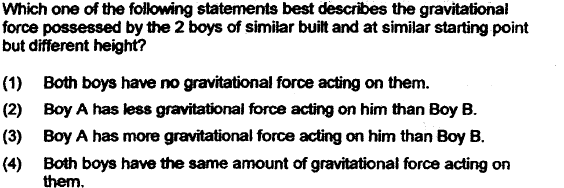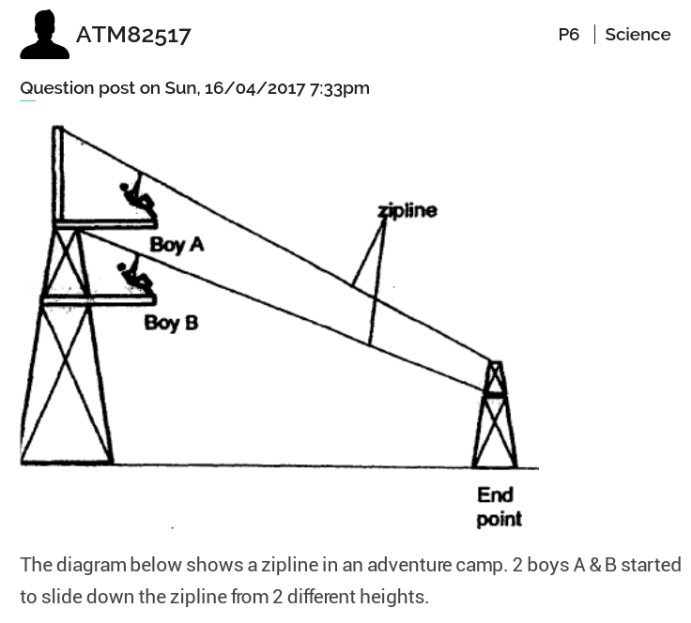# QuestionMy question is why both boys have the same amount of gravitational force acting on them? thank you.I can’t find reference in primary science guide that P6 needs to learn gravitatiional force. F=mg (m is mass of object and g is gravitational acceleration, constant, for free falling object) . So for falling objects of same mass, downward gravitational force is the same.

The question is likely a trick question to confuse a student between

(1) graviational potential energy (more for the boy A)-in syllabus and

(2) gravitational force (same for both boy).

If concept of g is not required, then this question is invalid to test a P6 student. Can consider double check with school science teacher. good if you can let us know here any outcome or reference point. Thanks.

2 Replies 0 Likes

Agree, when you talk about force you talk about mass and acceleration (formula wise, they ar both factors of force). Since both boys are similar built, i.e. mass is the same for both, and the acceleration is also fixed at 9.81 m/sec2 . So the gravitational FORCE (.e. the weight of the boys) is the same…

one big round is actually talking about the weight(not mass) of the boys in NEWTON…